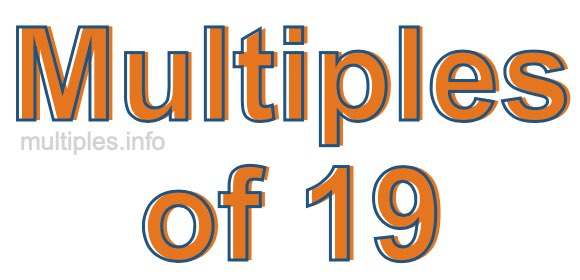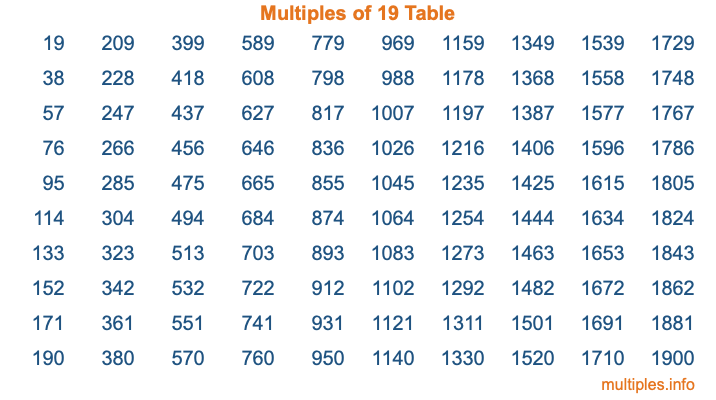Multiples of 19Welcome to the Multiples of 19 page. Here we will first teach you everything you will ever need to know about the multiples of 19, and then give you a study guide summary of everything we taught you to make sure you remember it all. Use this page to look up facts and learn information about the multiples of 19. This page will make you a multiples of nineteen expert!

Definition of Multiples of 19
Multiples of 19 are all the numbers that when divided by 19 equal an integer. Each of the multiples of 19 are called a multiple. A multiple of 19 is created by multiplying 19 by an integer.

Therefore, to create a list of multiples of 19, you start with 1 multiplied by 19, then 2 multiplied by 19, then 3 multiplied by 19, and so on for as long as you want. Thus, the list of the first five multiples of 19 is 19, 38, 57, 76, and 95. To see a larger list of multiples of 19, see the printable image of Multiples of 19 further down on this page. We also have a category where you can choose any nth multiple of 19.

Multiples of 19 Checker
The Multiples of 19 Checker below checks to see if any number of your choice is a multiple of 19. In other words, it checks to see if there is any number (integer) that when multiplied by 19 will equal your number. To do that, we divide your number by 19. If the the quotient is an integer, then your number is a multiple of 19.

Is  a multiple of 19?

Least Common Multiple of 19 and ...
A Least Common Multiple (LCM) is the lowest multiple that two or more numbers have in common. This is also called the smallest common multiple or lowest common multiple and is useful to know when you are adding our subtracting fractions. Enter one or more numbers below (19 is already entered) to find the LCM.

Check out our LCM Calculator if you need more details about the Least Common Multiple or if you need the LCM for different numbers for adding and subtraction fractions.

nth Multiple of 19
As we stated above, 19 is the first multiple of 19, 38 is the second multiple of 19, 57 is the third multiple of 19, and so on. Enter a number below to find the nth multiple of 19.

th multiple of 19

Multiples of 19 vs Factors of 19
19 is a multiple of 19 and a factor of 19, but that is where the similarities end. All postive multiples of 19 are 19 or greater than 19. All positive factors of 19 are 19 or less than 19.

Below is the beginning list of multiples of 19 and the factors of 19 so you can compare:

Multiples of 19: 19, 38, 57, 76, 95, etc.

Factors of 19: 1, 19

As you can see, the multiples of 19 are all the numbers that you can divide by 19 to get a whole number. The factors of 19, on the other hand, are all the whole numbers that you can multiply by another whole number to get 19.

It's also interesting to note that if a number (x) is a factor of 19, then 19 will also be a multiple of that number (x).

Multiples of 19 vs Divisors of 19
The divisors of 19 are all the integers that 19 can be divided by evenly. Below is a list of the divisors of 19.

Divisors of 19: 1, 19

The interesting thing to note here is that if you take any multiple of 19 and divide it by a divisor of 19, you will see that the quotient is an integer.

Multiples of 19 Table
Below is an image of the first 100 multiples of 19 in a table. The table is in chronological order, column by column. The first column has the first ten multiples of 19, the second column has the next ten multiples of 19, and so on.The Multiples of 19 Table is also referred to as the 19 Times Table or Times Table of 19. You are welcome to print out our table for your studies.

Negative Multiples of 19
Although not often discussed or needed in math, it is worth mentioning that you can make a list of negative multiples of 19 by multiplying 19 by -1, then by -2, then by -3, and so on, to get the following list of negative multiples of 19:

-19, -38, -57, -76, -95, etc.

Multiples of 19 Summary
Below is a summary of important Multiples of 19 facts that we have discussed on this page. To retain the knowledge on this page, we recommend that you read through the summary and explain to yourself or a study partner why they hold true.

There are an infinite number of multiples of 19.

A multiple of 19 divided by 19 will equal a whole number.

19 divided by a factor of 19 equals a divisor of 19.

The nth multiple of 19 is n times 19.

The largest factor of 19 is equal to the first positive multiple of 19.

19 is a multiple of every factor of 19.

19 is a multiple of 19.

A multiple of 19 divided by a divisor of 19 equals an integer.

19 divided by a divisor of 19 equals a factor of 19.

Any integer times 19 will equal a multiple of 19.

Multiples of a Number
Here you can get the multiples of another number, all with the same attention to detail as we did for multiples of 19 on this page.

Multiples of
Multiples of 20
Did you find our page about multiples of nineteen educational? Do you want more knowledge? Check out the multiples of the next number on our list!